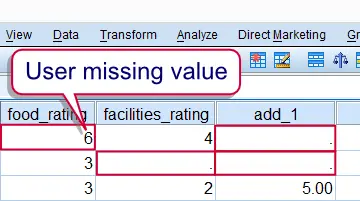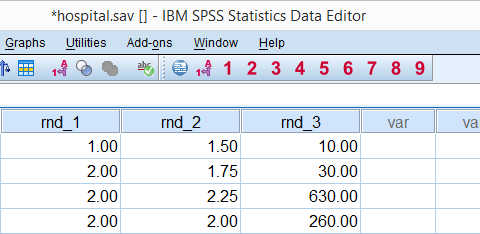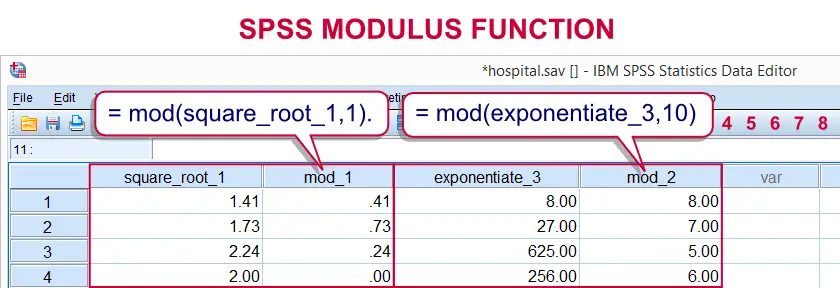# SPSS tutorials

BASICS DATA ANALYSIS T-TEST ANOVA CHI-SQUARE TEST

# SPSS Main Numeric Functions

This tutorial covers some obvious and some less obvious functions for editing numeric variables. You can follow along with this tutorial by downloading and opening hospital.sav. They syntax we'll use can be copy-pasted or downloaded here.## Specifying User Missing Values

This tutorial will only use the last 2 variables in the data.These are ordinal variable so, strictly, calculations are not allowed on them. However, this is common practice nevertheless as explained in Assumption of Equal Intervals. Before doing anything else, we first specify 6 as a user missing value by running the line of syntax below.

*Specify 6 as user missing value for food_rating and facilities_rating.

missing values food_rating facilities_rating (6).

Numeric values can be added in SPSS by simply using the + operator. The syntax below gives an example by computing add_1 as the addition of food_rating and facilities_rating

compute add_1 = food_rating + facilities_rating.
exe.

One thing to keep in mind is that if any of the operands (mostly “input variables”) is a missing value, the result will a system missing value. This can be avoided by using the `SUM` function instead, which we'll discuss later.Valid + Missing Value = Missing Value

## SPSS Subtractions

Subtracting numeric values in SPSS is straightforward and can be done with the - operator as shown in the syntax below.

*Subtract food_rating from facilities_rating.

compute sub_1 = facilities_rating - food_rating.
exe.

## SPSS Multiplications

Numeric values can be multiplied in SPSS with the * operator. The syntax below demonstrates this.

*Multiply food_rating with _facilities_rating.

compute multiply_1 = food_rating * facilities_rating.
exe.

## SPSS Divisions

Simply use the / operator for division in SPSS. The syntax below gives an example.

*Divide food_rating by _facilities_rating.

compute divide_1 = food_rating / facilities_rating.
exe.

## SPSS Exponentiations

Exponentiating in SPSS is done by the ** operator. The syntax below gives some examples. Note that you can also compute (square or other) roots by exponentiation as shown in the second example.

*1. Square food_rating.

compute exponentiate_1 = food_rating **2.
exe.

*2. Compute cubic root of food_rating.

compute exponentiate_2 = food_rating **(1/3).
exe.

*3. Raise food_rating to the power facilities_rating.

compute exponentiate_3 = food_rating ** facilities_rating.
exe.

## SPSS SQRT Function

Computing square roots in SPSS can be done by exponentiating a number to the power 0.5 as hinted at by the previous syntax example. For the less mathematically inclined, SPSS also has the `SQRT` function. For the sake of completeness, we'll demonstrate it below.

*Compute square root of food_rating.

compute square_root_1 = sqrt(food_rating).
exe.

## SPSS RND Function

Rounding numbers in SPSS is done with the `RND` function. `RND` takes an optional second argument, which is the nearest value to round to. Like so, you can round to the nearest quarter point (second example below) or tenfold (third example).
If the second argument is omitted, values will be rounded to the nearest integer (first example). Note that the syntax below uses some variables that were computed in the previous examples.SPSS RND function can round values to integers, quarter points and tenfolds

## SPSS RND Syntax Examples

*1. Round square_root_1 to nearest integer.

compute rnd_1 = rnd(square_root_1).
exe.

*2. Round square_root_1 to nearest quarter value.

compute rnd_2 = rnd(square_root_1,.25).
exe.

*3. Round exponentiate_3 to nearest tenfold.

compute rnd_3 = rnd(exponentiate_3,10).
exe.

## SPSS TRUNC Function

Truncating numbers basically means rounding them down to the nearest integer or other value. SPSS includes a `TRUNC` function for doing so. It takes an optional second argument, which is the nearest value to which to truncate. Like so, you may truncate a number to the nearest tenfold (third example below) are quarter value (second example).
If the second argument is omitted, numbers will be truncated to the nearest integer. Note that the syntax below uses some variables that were computed in the previous examples.

## SPSS TRUNC Syntax Examples

*1. Trunc square_root_1 to nearest integer.

compute trunc_1 = trunc(square_root_1).
exe.

*2. Truncate square_root_1 to nearest quarter value.

compute trunc_2 = trunc(square_root_1,.25).
exe.

*3. Truncate exponentiate_3 to nearest tenfold.

compute trunc_3 = trunc(exponentiate_3,10).
exe.

## SPSS MOD Function

The modulus function returns the remainder of a number after subtracting a second number from it as many times as possible. Note that it's basically the opposite of truncating; instead of throwing away the remainder, we now throw away everything except for the remainder.
In SPSS, `MOD(a,b)`, means “substract b from a as many times as possible and return the remainder of a”. If that still sounds a little abstract, try running the two examples in the syntax below. Note that it uses some variables from the previous examples. The results are also shown in the screenshot that follows.

## SPSS MOD Syntax Examples

*1. Extract the decimals of square_root_1.

compute mod_1 = mod(square_root_1,1).
exe.

*2. Remove all tenfolds of exponentiate_3.

compute mod_2 = mod(exponentiate_3,10).
exe.# Let me know what you think!

*Required field. Your comment will show up after approval from a moderator.

# This tutorial has 9 comments

•### By Maysam Fadaei-kenarsary on November 1st, 2019

•### By PATRICK KOOMSON on October 18th, 2019

This is my goto website anytime I need some quick reference. I have bookmarked the page.
Thank you very much.

•### By Vishal on October 11th, 2019

•